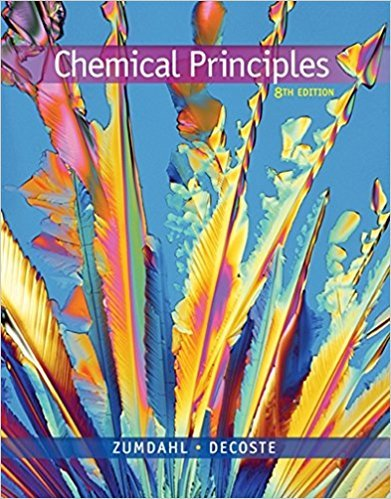×

×

# Solutions for Chapter 14: Orbitals## Full solutions for Chemical Principles | 8th Edition

ISBN: 9781305581982Solutions for Chapter 14: Orbitals

Solutions for Chapter 14
4 5 0 371 Reviews
30
0
##### ISBN: 9781305581982

This textbook survival guide was created for the textbook: Chemical Principles, edition: 8. Since 101 problems in chapter 14: Orbitals have been answered, more than 80548 students have viewed full step-by-step solutions from this chapter. This expansive textbook survival guide covers the following chapters and their solutions. Chemical Principles was written by and is associated to the ISBN: 9781305581982. Chapter 14: Orbitals includes 101 full step-by-step solutions.

Key Chemistry Terms and definitions covered in this textbook
• a-Amino acid

An amino acid in which the amino group is on the carbon adjacent to the carboxyl group.

• alkoxide

The conjugate base of an alcohol.

• cathode

An electrode at which reduction occurs. (Section 20.3)

• chemical property.

Any property of a substance that cannot be studied without converting the substance into some other substance. (1.6)

• continuous spectrum

A spectrum that contains radiation distributed over all wavelengths. (Section 6.3)

• deshielded

In NMR spectroscopy,protons or carbon atoms whose surrounding electron density is poor.

• E1

A unimolecular elimination reaction.

• Frost circles

A simple method for drawing the relative energy levels of the MOs for a ring assembled from continuously overlapping p orbitals.

• geometric isomerism

A form of isomerism in which compounds with the same type and number of atoms and the same chemical bonds have different spatial arrangements of these atoms and bonds. (Sections 23.4 and 24.4)

• Homotopic groups

Atoms or groups on an atom that give an achiral molecule when one of the groups is replaced by another group. The hydrogens of the CH2 group of propane, for example, are homotopic. Replacing either one of them with deuterium gives 2-deuteropropane, which is achiral. Homotopic groups have identical chemical shifts under all conditions

• hypothesis

A tentative explanation of a series of observations or of a natural law. (Section 1.3)

Radiation that has sufficient energy to remove an electron from a molecule, thereby ionizing it. (Section 21.9)

• l amino acid

Amino acids with Fischer projections that resemble the Fischer projections of l sugars.

• methyl shift

A type of carbocation rearrangement in which a methyl group migrates.

• parts per million (ppm)

The concentration of a solution in grams of solute per 106 (million) grams of solution; equals milligrams of solute per liter of solution for aqueous solutions. (Section 13.4)

• Polar covalent bond

A covalent bond between atoms whose difference in electronegativity is between approximately 0.5 and 1.9.

• pressure

A measure of the force exerted on a unit area. In chemistry, pressure is often expressed in units of atmospheres (atm) or torr: 760 torr = 1 atm; in SI units pressure is expressed in pascals (Pa). (Section 10.2)

• Shell

A region of space around a nucleus that can be occupied by electrons, corresponding to a principal quantum number

• sp2-hybridized orbitals

Atomic orbitals that are achieved by mathematically averaging one s orbital with two p orbitals to form three hybridized atomic orbitals.

• Tollens’ reagent

A solution prepared by dissolving Ag2O in aqueous ammonia; used for selective oxidation of an aldehyde to a carboxylic acid.